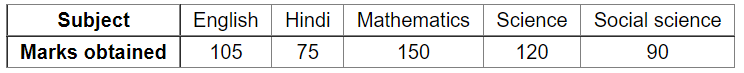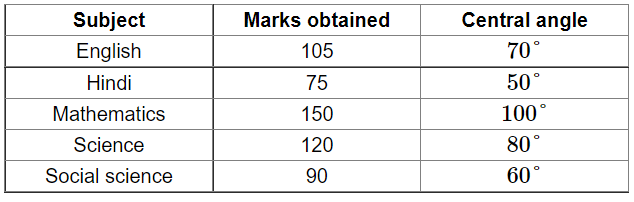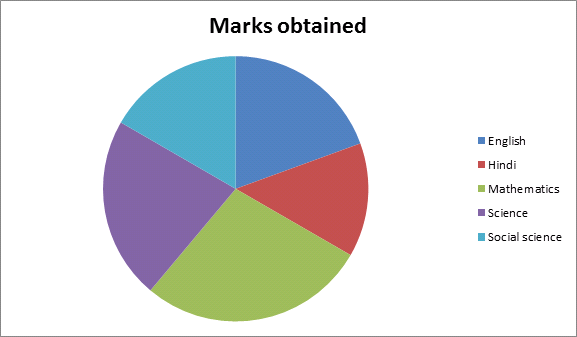# The marks obtained by Sudhir in an examination are given below:

Question:

The marks obtained by Sudhir in an examination are given below:Represent the above data by a pie chart.

Solution:

Total marks obtained $=(105+75+150+120+90)=540$

Central angle of each subject $=\left(\frac{\text { marks obtained in each subject }}{\text { total marks obtained }} \times 360\right)^{\circ}$

Calculation of central anglesConstruction of pie chart

Steps of construction:

1. Draw a circle of any convenient radius.

2. Draw a horizontal radius of this circle.

3. Draw sectors whose central angles are $70^{\circ}, 50^{\circ}, 100^{\circ}, 80^{\circ}$ and $60^{\circ}$.

4. Shade the sectors so obtained differently and label each one of them.

Thus, we obtain the required pie chart as shown in the figure below.